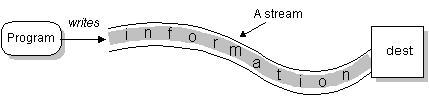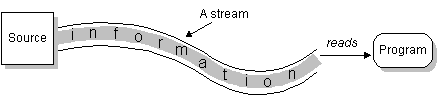# PIC10A Lecture 3

1.2,1.3,1.4

## Input and Output Stream

A stream is a flow of information.

### Output Stream

Output Stream is a stream of output generated by the program. e.g. to screen### cout

• use `cout <<`
• stands for console output
• basically like "print this to screen"
• as usual, the statments must end in semicolon (;)

### Examples

basic example
 `cout << "Hello! PIC10A students.";`
Here is the output
 Hello! PIC10A students.

You can link 2 or more sentences together.
 ```cout << "Hello! " << "PIC10A students. " << "Welcome to UCLA.";```
This is same as
 ```cout << "Hello! "; cout << "PIC10A students. "; cout << "Welcome to UCLA. ";```
The output is
 Hello! PIC10A students. Welcome to UCLA.

You can include variables
 ```int appleNum = 5; cout << "I ate " << appleNum << " apples this morning.";```
The output is
 `I ate 5 apples this morning.`
`appleNum` is substituted by its value (5).

You can include arithmetic expressions.
 ```int speed = 45, time = 2; cout << "I drove " << (speed*time) << " miles.";```
Here is the output
 `I drove 90 miles.`
The expression `speed*time` is evaluated. The value is then printed.
It is better the put parentheses (but not necessary in Visual C++)  around the expresion.

### Escape Sequences

• some characters present difficulties
• example, think about the quote marks "
• You can `cout` special characters using "escape sequences"
• A backslash, \, preceding a character tells the compiler that the sequence following the backslash does not have the same meaning as the character appearing by itself. Such a sequence is called an escape sequence.
Sequence
Meaning
```\n ``` New line
```\t ``` tab
```\a ``` alert(sounds the alert noise), typically a bell
```\\ ``` Backslash (allows you to place a backslash in a quoted expression)
```\" ``` Double quote(mostly used to place a double quote inside a quoted string)
```\' ``` Double quote(mostly used to place a single quote inside single quotes)

### Examples

Example
 ```int num1 = 10, num2 = 20; cout << "The first number is " << num1      << ".\nThe second number is " << num2  << ".\n";```
Output
 The first number is 10. The second number is 20.

Example
 ```cout << "Backslash \\ is used in escape sequences.\n";```
Output
 `Backslash \ is used in escape sequences.`

Example
 ```cout<<"\"PIC10A is cool,\" Mary said.\n";```
Output
 `"PIC10A is cool," Mary said.`

### endl vs "\n"

• `endl` represnets "end line"
• It is same as `"\n"`;
 `cout << "Hello World!\n";`
is same as
 `cout << "Hello World!" << endl;`

### Formatting for Numbers with a Decimal Point

Example
 ```cout<<"1/11 is "<< 1./11. << endl;```
Output
 1/11 is 0.0909091

Here is the "magic formula". It causes the number to be displayed with the exact number of digits after the decimal point.
 ```#include using namespace std; int main() {     cout.setf(ios::fixed);     cout.setf(ios::showpoint);     cout.precision(2);        cout<<"1/11 is "<< 1./11. << endl;     return 0; }```
Output
 `1/11 is 0.09`
Exercise:  Use `cout.precision(3)` in the program, what is the output?``` ```

### Input Stream

Input stream is info fed to the computer for the program.• `cin >> variable;` or cin >> variable1>> variable2...;
• stands for "console input"
• Like "read into variables"
• when a program reaches a cin statement, it waits for input to be entered from the keyboard.
• it sets the first variable equal to the first value typed at the keyboard, the second variable equal to the second value typed....
• number in the input must be seperated one or more space or by a line break (return).

• make your program more readable and easier to modify.
• there are 2 types of comment.
• you can put comments anywhere in the program.
• compilers ignore all the comments. Comments are just for human. It has no effect on programs.
• if something is important and not obvious, it merits a comment.
Example
 ```/* PIC 10A Sample Program Written by Charles Li The program asks the user to input the number of quarters, dimes, nickels and output the total value of all the coins */ #include using namespace std; int main() {     double quarterValue = 0.25, dimeValue = 0.1, nickelValue = 0.05;     int numOfQuarters, numOfDimes, numOfNickels;     double value; //value of all the coins     cout << "Input the number of quarters, dimes and nickels.\n";     // ask the user to input the numbers     cin >> numOfQuarters >> numOfDimes >> numOfNickels;     value = quarterValue*numOfQuarters            + dimeValue*numOfDimes            + nickelValue*numOfNickels;     cout << "The value is " << value <<".\n";       return 0; }```

### Single line comments

 `// your comment`
All the text between the `//` and the end of the line is a comment

 /* your comment line 1 your comment line2 your comment line 3 */
• All the text between `/*` and `*/` are comments
• line break is allowed

## More about types

### float and double

• floating-point notation, example `double x = 3.568902;`
• scientific notation, example ```double a = 2.3e-5```;(it means 2.3 times 10-5, or 0.000023)

### char

• represents a single character (like letters or  punctuation marks)
• Use single quotes to initalize or assingn : example ```char ch = 'k';```
• different from strings. ("Hello world" is a string, "A" is a string, because they are enclosed in double quotes)
• can only hold 1 character.

### bool

• means `true` or `false`
• example: `bool billPaid = false;`

## Arithmetic Operations and Expressions

• C++ allows you to form expresions using variables, and arithmetic operators: `+,-,*, /.`

### Order of operations in expressions

• Expressions are evaluated left to right
• whatever is in` ( ) `is first
• next is `* ` or` /`.
• finally, `+` or `-`

### Mixed types

• all the arithmetic operators can be used with the number of type `int` , type `double`, or one number of each type.
• if both numbers are integers, the result is integer
• if one of them is double, the result is double
Question
1) What is the type of `totalWork` in below?
```int workDays = 5; double hours = 7.5; ?????  totalWork = workDays*hours; ```2) What is the return type if a float is added to a double?
3) What is the output of the following program
```int a = 7, b = 3; cout << a/b <<endl; ```

### The % operator

• % operator gives the remainder of the division of two integers
• e.g. `cout << 8%3 << endl;` is 2

### Shorthand

examples
shorthand
meaning
```a+=1; ``` ```a = a+1; ```
```b-=3.5; ``` ```b = b-3.5; ```
```c*=2; ``` ```c = c*2; ```
```d/=3.1415; ``` ```d = d/3.1415; ```
```e++ ( or ++e) ``` ```e = e+1;   ```
```f-- ( or --f) ``` ```f = f-1; ```

Difference between `x++ `and ```++x ```
Code Output ```#include using namespace std; int main() {     int a = 5;     int b = a++;     cout << b < using namespace std; int main() {     int a = 5;     int b = ++a;     cout << b <